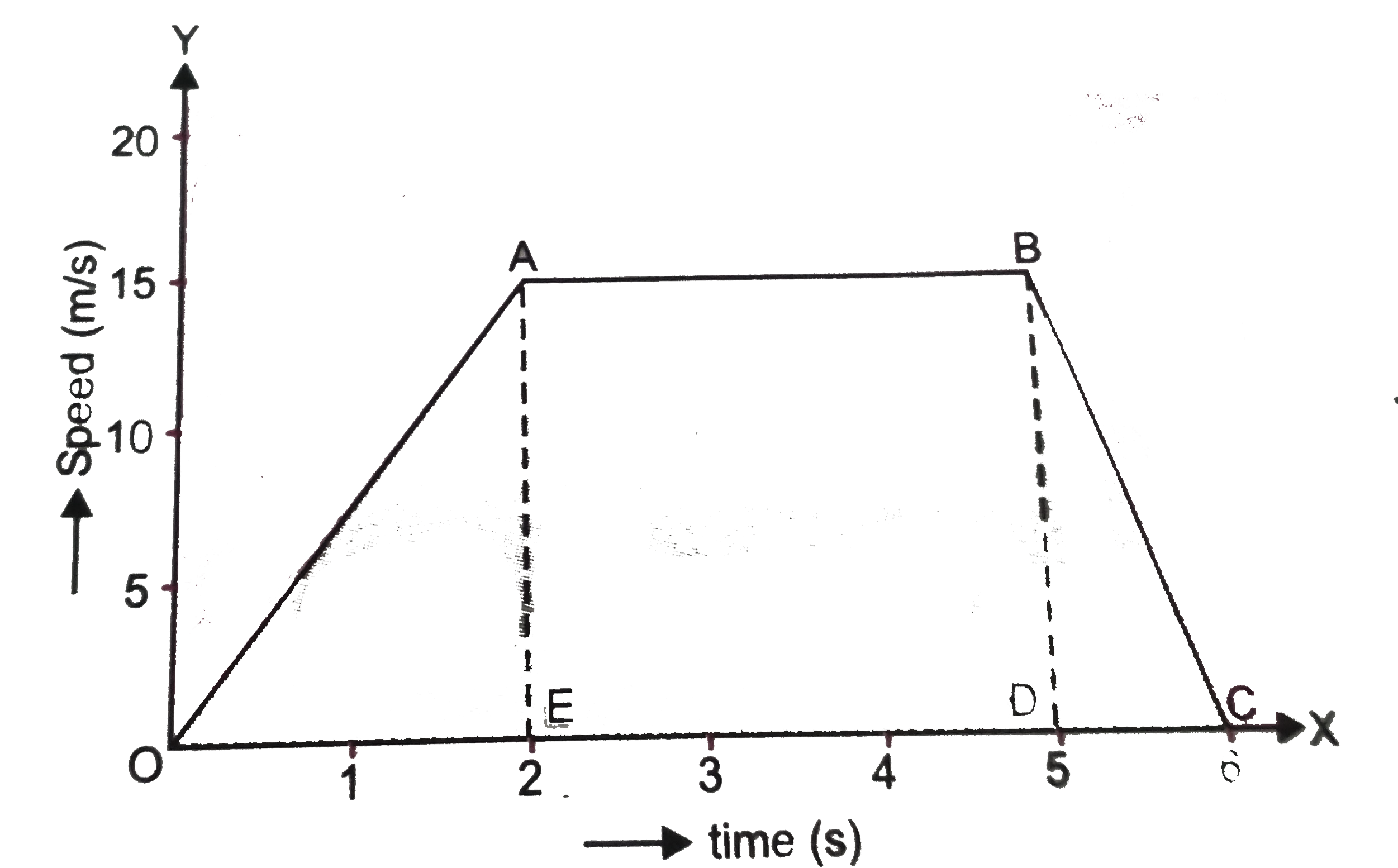Question

Updated on:30/05/2023

# The speed- time graph of a car is given in (figure) The car weights 1000kg (a) What is the distance travelled by the car in first two seconds? (b) What is the braking force applied at the end of 5seconds to bring the car to a stop within one second?Text Solution

Solution
(i) We will first calculate the distance travelled in the first two seconds. From the given graph we find that :
Distance travelled in first two seconds = Area under line AB and the time axis
=Area of triangle AB2
=12×base×Height
=12×2×15
=15 m
Thus, the distance travelled by the car in the first two seconds is 15 metres.
(ii) The braking force is to be calculated by using the formula
Force=mass×acceleration
or F=m×a
Here, the mass of the car is given as 1000 kg but the acceleration in the last one second (from point C to point D) is to be obtained from the graph shown above. Now, we know that the acceleration is given by the slope of speed-time graph. So,
Acceleration in last one second = Slope of line CD
=PerpendicularBase
=151
=15m/s2
If we look at the above given graph, we will find that the speed of the car is decreasing from point C to point D. That is, the acceleration from C to D is negative and hence it should be written with a minus sign. Thus,
Acceleration, a =-15 m/s2
Now,putting m= 1000 kg and a =15 m/s2 in formula (1), we get:
Force,F=1000 × (-15)
=-15000N
Thus, the force applied by the brakes to stop the car is 15000 newtons. The negative sign of force here shows that the force is being applied in a direction opposite to the motion of the car. That is, it is a retarding force.